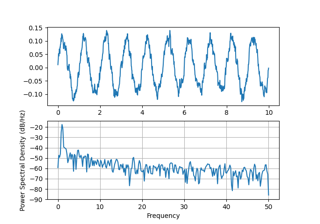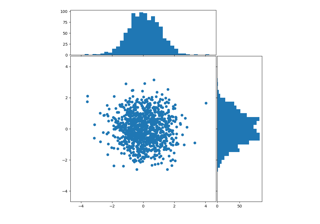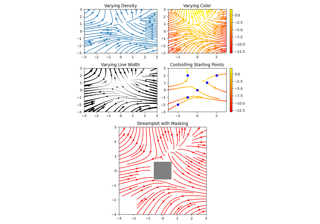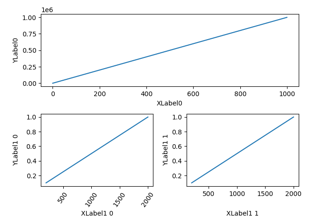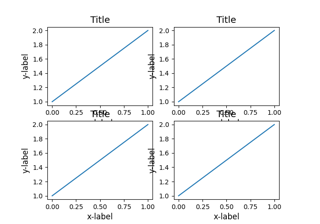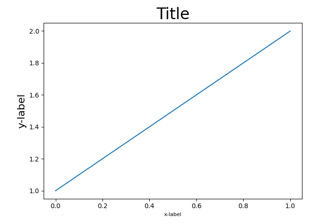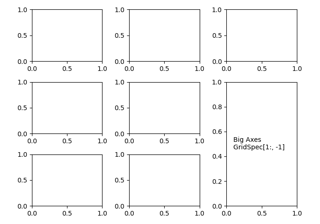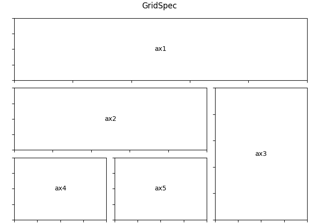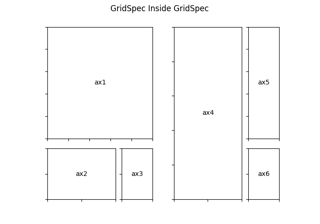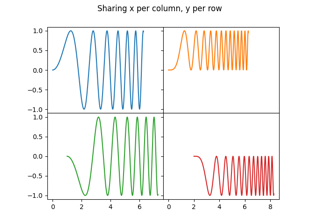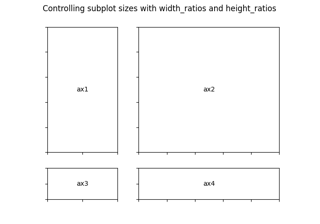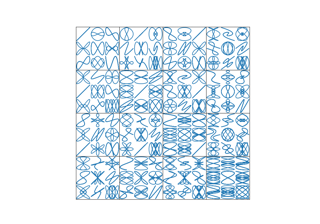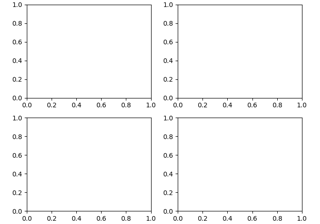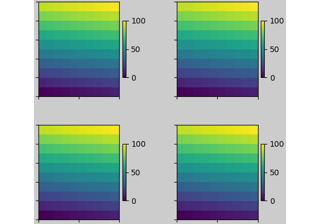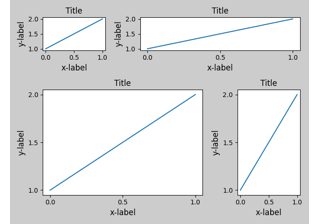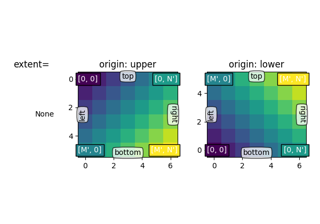# matplotlib.gridspec.GridSpec¶

class matplotlib.gridspec.GridSpec(nrows, ncols, figure=None, left=None, bottom=None, right=None, top=None, wspace=None, hspace=None, width_ratios=None, height_ratios=None)[source]

A grid layout to place subplots within a figure.

The location of the grid cells is determined in a similar way to SubplotParams using left, right, top, bottom, wspace and hspace.

Parameters: nrows, ncolsintThe number of rows and columns of the grid. figureFigure, optionalOnly used for constrained layout to create a proper layoutbox. left, right, top, bottomfloat, optionalExtent of the subplots as a fraction of figure width or height. Left cannot be larger than right, and bottom cannot be larger than top. If not given, the values will be inferred from a figure or rcParams at draw time. See also GridSpec.get_subplot_params. wspacefloat, optionalThe amount of width reserved for space between subplots, expressed as a fraction of the average axis width. If not given, the values will be inferred from a figure or rcParams when necessary. See also GridSpec.get_subplot_params. hspacefloat, optionalThe amount of height reserved for space between subplots, expressed as a fraction of the average axis height. If not given, the values will be inferred from a figure or rcParams when necessary. See also GridSpec.get_subplot_params. width_ratiosarray-like of length ncols, optionalDefines the relative widths of the columns. Each column gets a relative width of width_ratios[i] / sum(width_ratios). If not given, all columns will have the same width. height_ratiosarray-like of length nrows, optionalDefines the relative heights of the rows. Each column gets a relative height of height_ratios[i] / sum(height_ratios). If not given, all rows will have the same height.
__getstate__(self)[source]
__init__(self, nrows, ncols, figure=None, left=None, bottom=None, right=None, top=None, wspace=None, hspace=None, width_ratios=None, height_ratios=None)[source]
Parameters: nrows, ncolsintThe number of rows and columns of the grid. figureFigure, optionalOnly used for constrained layout to create a proper layoutbox. left, right, top, bottomfloat, optionalExtent of the subplots as a fraction of figure width or height. Left cannot be larger than right, and bottom cannot be larger than top. If not given, the values will be inferred from a figure or rcParams at draw time. See also GridSpec.get_subplot_params. wspacefloat, optionalThe amount of width reserved for space between subplots, expressed as a fraction of the average axis width. If not given, the values will be inferred from a figure or rcParams when necessary. See also GridSpec.get_subplot_params. hspacefloat, optionalThe amount of height reserved for space between subplots, expressed as a fraction of the average axis height. If not given, the values will be inferred from a figure or rcParams when necessary. See also GridSpec.get_subplot_params. width_ratiosarray-like of length ncols, optionalDefines the relative widths of the columns. Each column gets a relative width of width_ratios[i] / sum(width_ratios). If not given, all columns will have the same width. height_ratiosarray-like of length nrows, optionalDefines the relative heights of the rows. Each column gets a relative height of height_ratios[i] / sum(height_ratios). If not given, all rows will have the same height.
__module__ = 'matplotlib.gridspec'
get_subplot_params(self, figure=None)[source]

Return the SubplotParams for the GridSpec.

In order of precedence the values are taken from

• non-None attributes of the GridSpec
• the provided figure
• rcParams["figure.subplot.*"]
locally_modified_subplot_params(self)[source]

Return a list of the names of the subplot parameters explicitly set in the GridSpec.

This is a subset of the attributes of SubplotParams.

tight_layout(self, figure, renderer=None, pad=1.08, h_pad=None, w_pad=None, rect=None)[source]

Adjust subplot parameters to give specified padding.

Parameters: padfloatPadding between the figure edge and the edges of subplots, as a fraction of the font-size. h_pad, w_padfloat, optionalPadding (height/width) between edges of adjacent subplots. Defaults to pad. recttuple of 4 floats, default: (0, 0, 1, 1), i.e. the whole figure(left, bottom, right, top) rectangle in normalized figure coordinates that the whole subplots area (including labels) will fit into.
update(self, **kwargs)[source]

Update the subplot parameters of the grid.

Parameters that are not explicitly given are not changed. Setting a parameter to None resets it to rcParams["figure.subplot.*"].

Parameters: left, right, top, bottomfloat or None, optionalExtent of the subplots as a fraction of figure width or height. wspace, hspacefloat, optionalSpacing between the subplots as a fraction of the average subplot width / height.

## Examples using matplotlib.gridspec.GridSpec¶